QuestionAnswers

# Find median of following data.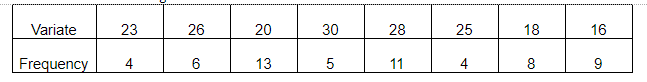Hint: Median- The median is the middle number is an ordered set of data.
In a frequency table, the observations are already arranged in an ascending order. We can obtain the median by looking for the value in the middle position.
If there is an odd number of observations, the median is the middle number.
If there is an even number of observations, the median will be the mean of the two central numbers.
1. When number of observations $(n)$ is odd, median is ${\left( {\dfrac{{n + 1}}{2}} \right)^{th}}$ position
2. When number of observation $(n)$ is even, then median is average of value at ${\left( {\dfrac{n}{2}} \right)^{th}}$ and ${\left( {\dfrac{{n + 2}}{2}} \right)^{th}}$ position.
i.e., $\boxed{\dfrac{{{{\left( {\dfrac{n}{2}} \right)}^{th}} + {{\left( {\dfrac{{n + 2}}{2}} \right)}^{th}}}}{2}}$
Above question has an even number N $=$ even number.
So we will learn this method in our solution.
Let's take an example for n $=$ odd number.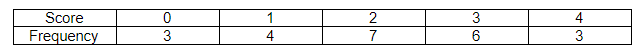Here $N = \Sigma fi = 3 + 4 + 7 + 6 + 3$
$= 23$
So, median will be ${\left( {\dfrac{{23 + 1}}{2}} \right)^{th}}$ position ${\left( {\dfrac{{24}}{2}} \right)^{th}}$ position value.
On 12th position value
But for this we will find cumulative frequency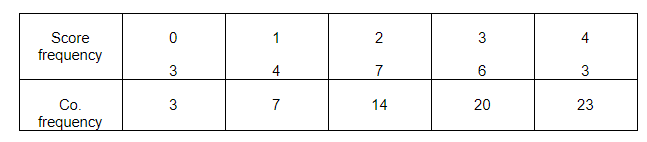Value at position 12th is 2.
So median $=$ 2

Complete step by step solution: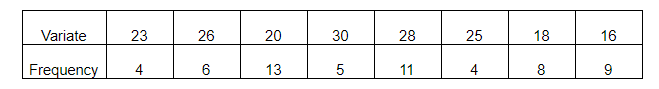Total frequency i.e., $\sum\limits_{i = 1}^8 {fi = 4 + 6 + 13 + 5 + 11 + 4 + 8 + 9}$
$\sum\limits_{i = 1}^8 {fi = 60}$
$\Sigma fi = 60$, which is an even number.
So, median is the average of values at ${\left( {\dfrac{n}{2}} \right)^{th}}$ and ${\left( {\dfrac{{n + 2}}{2}} \right)^{th}}$ positions.
When $n = \Sigma fi = 60$
So, ${\left( {\dfrac{{60}}{2}} \right)^{th}}$ position is 30th
and ${\left( {\dfrac{{60 + 2}}{2}} \right)^{th}}$ position is 31st
To find out values at 30th of 31st position. We will find cumulative frequency from given data.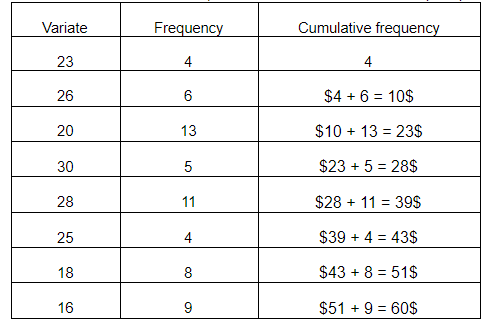From the above table we can easily conclude that variate at 30th position is 28, and variate at 31st position is also 28.
So, median $= \dfrac{{{{\left( {\dfrac{n}{2}} \right)}^{th}} + {{\left( {\dfrac{{n + 2}}{2}} \right)}^{th}}}}{2}$
$M = \dfrac{{28 + 28}}{2}$
$M = \dfrac{{56}}{2} = 28$
So, median M, of given data is 28

Note: When frequency is not given in question first we should arrange data in ascending or descending order.
Then,
Middle most number after arranging the given data is the median of given data.
For example- 7, 9, 12, 13, 16 middle most number after arranging the given data in ascending order is 12
So the median is 12.